### Paired Design

Define the following notation: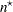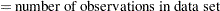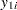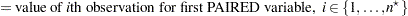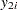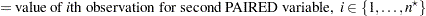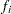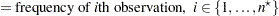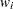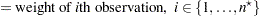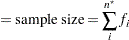#### Normal Difference (DIST=NORMAL TEST=DIFF)

The analysis is the same as the analysis for the one-sample design in the section Normal Data (DIST=NORMAL) based on the differences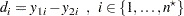#### Lognormal Ratio (DIST=LOGNORMAL TEST=RATIO)

The analysis is the same as the analysis for the one-sample design in the section Lognormal Data (DIST=LOGNORMAL) based on the ratios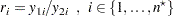#### Normal Ratio (DIST=NORMAL TEST=RATIO)

The hypothesis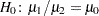, where
and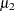are the means of the first and second PAIRED variables, respectively, can be rewritten as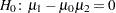. Thevalue and-value are computed in the same way as in the one-sample design in the section Normal Data (DIST=NORMAL) based on the transformed values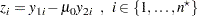Estimates and confidence limits are not computed for this situation.